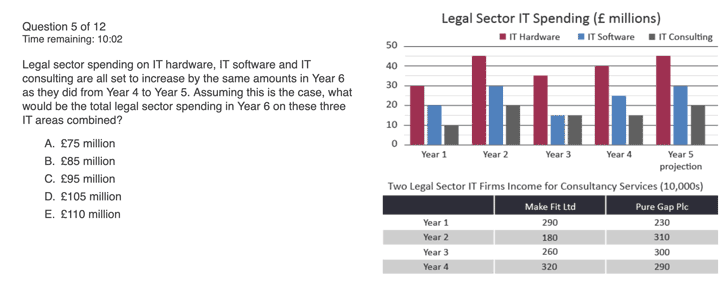⚡ Save 20% on Premium with code SAVE20

# SHL Numerical Reasoning Test: Format, Requirements & Preparation Tips###### Page contents:

Updated:

Note: AssessmentDay and its products are not affiliated with SHL. Our practice tests are intended only for candidate preparation, not for employee selection.

## SHL numerical reasoning tests

SHL is a leading global talent measurement company that offers a range of assessment tools and solutions to help organizations identify and develop top talent. SHL numerical reasoning tests are a type of cognitive ability test designed to assess a candidate's ability to understand and interpret numerical data, perform basic mathematical calculations, and solve problems. These tests are commonly used by organizations to evaluate a candidate's numerical reasoning and problem-solving skills, which are crucial in various roles and industries, such as finance, accounting, and business management.

## Format of the SHL numerical reasoning test

The format of the SHL numerical reasoning test typically includes multiple-choice questions that are presented in a timed format. The test usually lasts between 20 to 30 minutes and contains 20 to 30 questions, each with four possible answers. The questions are based on numerical data presented in tables, graphs, and charts, and the candidate is required to perform calculations and answer questions based on the data.

To give you an idea of a type of question, here is a screenshot from one of our numerical reasoning tests:## Don't leave your future career down to chance!

Get a completely free starter account

• Over 20 tests## How to prepare for SHL numerical tests

To prepare for the SHL numerical reasoning test, it is essential to have a good understanding of basic mathematical concepts, such as arithmetic, percentages, ratios, and basic algebra. Candidates should also practice solving problems quickly and accurately, as time is a significant factor in the test.

It is also recommended to familiarize oneself with the type of questions that may be included in the test. Some common question types include:

• Basic arithmetic: questions that test the candidate's ability to perform basic calculations, such as addition, subtraction, multiplication, and division.
• Percentage calculations: questions that require the candidate to perform calculations involving percentages, such as finding the percentage increase or decrease of a given value.
• Ratio and proportion: questions that require the candidate to work with ratios and proportions to find relationships between different values.
• Data interpretation: questions that require the candidate to understand and interpret numerical data presented in tables, graphs, and charts.
• Problem-solving: questions that require the candidate to use numerical data and perform calculations to solve real-world problems.

We have a guide to numerical reasoning formulas that you can study and use for your test.###### Key takeaways

To prepare for the SHL Numerical Reasoning Test, it is advisable to practice regularly with numerical reasoning tests and sample questions. This will help improve your speed and accuracy, as well as give you a better understanding of the types of questions that may be included in the test.

## Are SHL numerical reasoning tests hard?

The difficulty level of SHL Numerical Reasoning Tests can vary depending on the individual's mathematical skills and problem-solving ability. For some people, the tests may be relatively straightforward and easy to complete, while for others, they may present a significant challenge.

The tests assess a candidate's ability to understand and interpret numerical data, perform basic mathematical calculations, and solve problems. Therefore, individuals with strong mathematical skills and a good understanding of basic mathematical concepts are likely to find the tests easier to complete.

However, it's important to keep in mind that the tests are timed, and completing them within the allotted time frame can add an additional layer of difficulty. The pressure of the time constraint can make the test seem more challenging, even for individuals with strong numerical abilities.###### Did You Know

Practising mock tests can increase your test performance by over 50%

## Free practice numerical reasoning tests

###### Free Numerical Reasoning Test 1
Free

This free numerical reasoning test contains 12 questions and has a time limit of 12 minutes.

###### Free Numerical Reasoning Test 2
Free

This free numerical reasoning test contains 5 questions and has a time limit of 5 minutes.